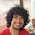### Give and Take

Source: Gazette of the Australian Mathematical Society

Problem: Two people, Give and Take, divide a pile of one hundred coins between themselves as follows. Give chooses a handful of coins from the pile and Take decides who will get them. This is repeated until all coins have been taken or until one of them has taken nine handfuls. In the latter case, the other person is allowed to take all of the remaining coins in the pile. What is the largest number of coins that Give can be sure of getting?

Update (05/02/10):
Solution: Solution by Aaditya Ramdas (CSE, IITB alumnus & Tower Research) in comments!! Further explained by me in comments!!

1.45.
Tell me if I can do any better.
But the simple strategy for Give should be to take 5 coins every time, which ensures that he gets 5x9=45 coins at least.

2.an even simpler strategy is to keep taking 6. if he starts taking all of them, he'll have 54, ull have 46.

3.actually u can show, by this very argument, thoda intuitively also, that the best strategy for Take is to take >=6, and give <=5. So ull see that 46 is the highest possible.

4.Ramdas (Ya, I am a Dastard)'s solution is correct. Its 46. :)

Strategy:
"Give" should always put aside 6 coins. If Take takes them, "Give" gets 100-54 = 46. If not, "Give" gets 54 :)

So, "Give" can ensure getting 46 coins always. Can he do better?

Suppose he follows any other strategy. Then "Take" will follow the strategy that he will take only if number of coins >= 6. And so, ensure that "Give" gets no more than 46 coins. So, this is the best strategy. :)

5.I think that there is a flaw in above solution. Suppose, that Give keeps taking the coins till 8 handfuls and then gives the last 6 coins to Give in that 9th handful. So, Give now has only 6 coins and Take has 94

My solution goes like this:

strategy : 1 1 1 1 1 1 1 1 46

Lemma: Suppose that after 8 handful coins have already been picked up, the number of coins to be picked up in the last go is half the remaining number of coins
Proof of Lemma: If he picks M s.t. M > R/2, where R is the remaining number of coins, then Take will take M. If he picked M s.t. M < R/2, then Take gives it to Give. In both the cases, the number of coins coming on Give's side is less than R/2 which was the case when M=R/2. Hence the lemma is proved.

Now, Give can always take the coins in first 8 handfuls. So, it is always best to give him the least and to save the maximum number of coins to be picked from in the last handfuls. So, this strategy is the best.

6.@Kapil,
I think that the question says that we stop after one person gets 9 handfuls, not after when total 9 handfuls have been picked.